# Why is copper smaller than zinc

## The redox series

To explain the redox series, let's first look at an interesting phenomenon: If we hold a zinc sheet in a copper sulfate solution, an elementary copper layer forms on the zinc sheet. But if we hold a sheet of copper in a zinc sulphate solution, no elemental zinc layer will form. We can even predict when such a response will work and when it will not. For this we need the electrochemical series of voltages (also called redox series).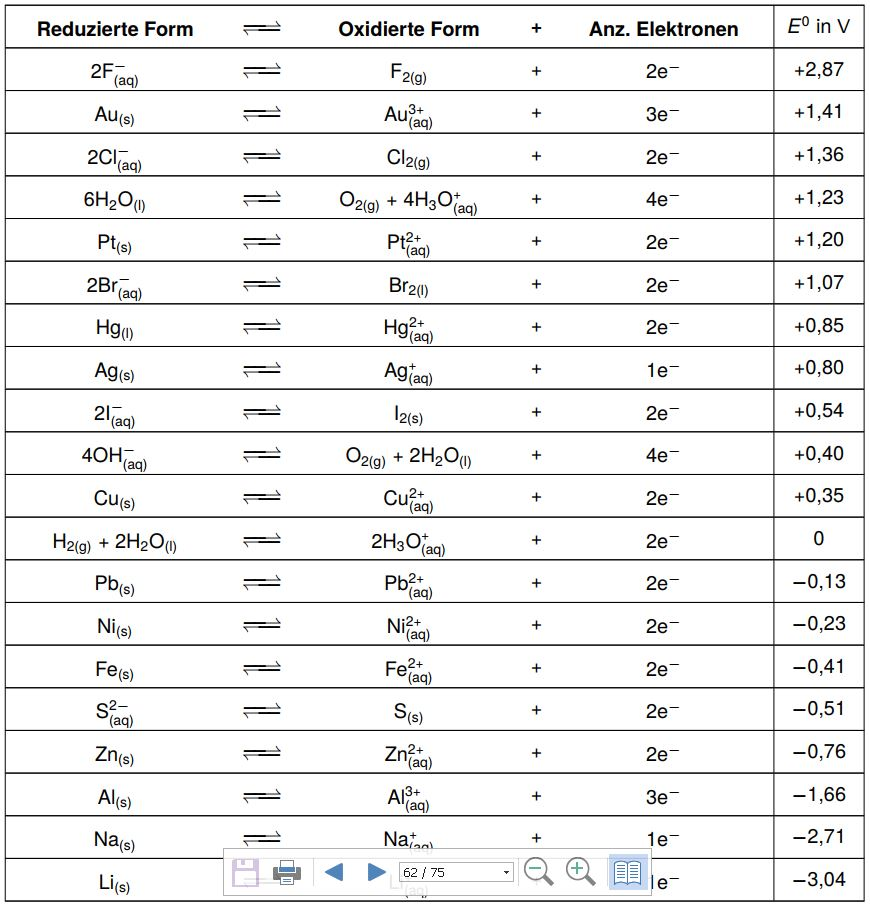Let's take a closer look at this electrochemical series. We have always given a corresponding redox couple and the corresponding number of electrons involved.

Let's look at copper: The reduced form in the table is Cu, the elemental copper. The oxidized form is Cu2 +. In order to convert these two into one another, two electrons have to be released or received. Now the number +0.35 is in the last column. This value is the so-called standard potential E0. In the following we have a very specific experimental set-up that is used to determine the standard potential: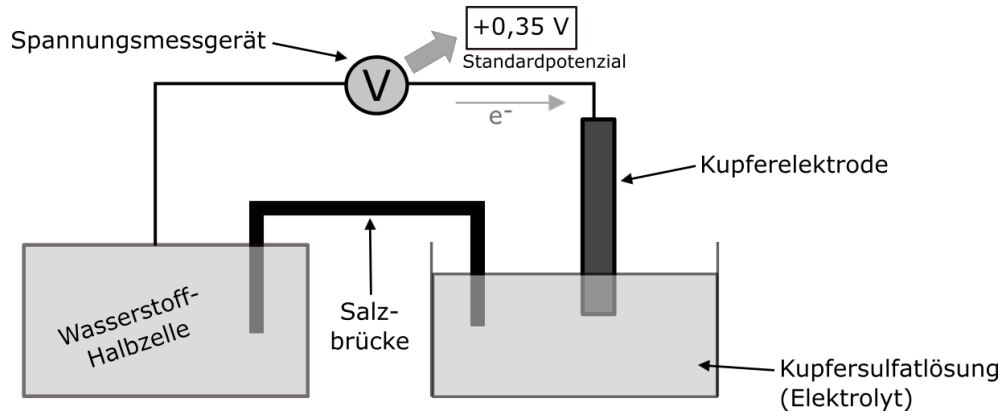We immerse a copper electrode in a copper sulfate solution so that a copper half-cell is formed. We connect the copper electrode to a hydrogen half-cell with a wire. In principle, this is exactly the same as the copper half-cell, with the difference that it has to be built up a bit differently, since hydrogen, unlike copper, is gaseous.

We now connect the two half-cells with a salt bridge. If we now connect a voltmeter, we can observe that a voltage of +0.35 V is present. That is exactly the value that is then also in the electrochemical series. Negative standard potentials now also appear in the voltage series. The sign of the standard potential indicates in which direction the electrons flow. With the copper half-cell we have a positive standard potential, that is, the electrons flow towards the copper half-cell.

We can observe a standard potential of −0.76 V for a zinc half-cell. In this case the electrons flow away from the zinc half-cell. We also say that zinc is a base metal because it likes to give up its electrons. Copper, on the other hand, is a noble metal because it does not like to give up its electrons and is therefore more likely to be in its elemental form.

For the electrochemical series, it should be noted that the values ​​for the standard potential apply to 1 molar solution. The values ​​also change at other concentrations.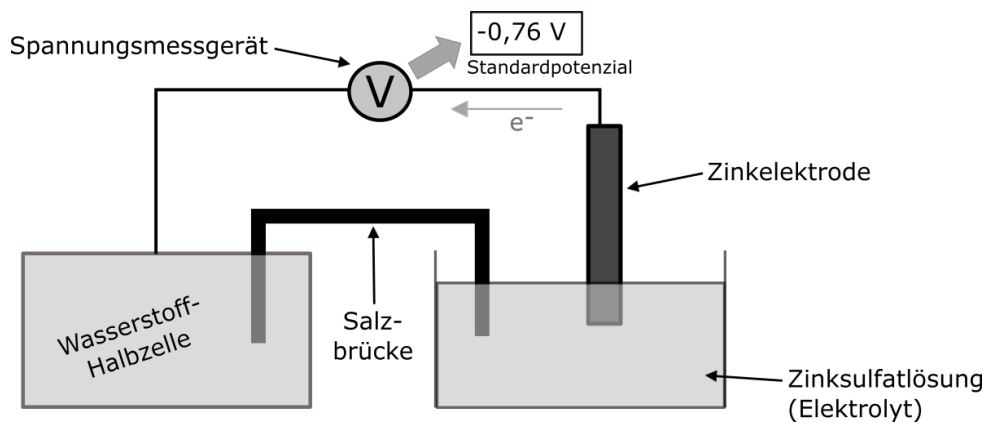Now we know what the values ​​in the table mean. Why do we need this?

Let's look again at the initial example with the zinc sheet in copper sulfate solution and the copper sheet in zinc sulfate solution. With the redox series we can predict in which variant the ions from the solution will react to form the elementary metal and in which variant not.

Let's look at copper and zinc in the redox series.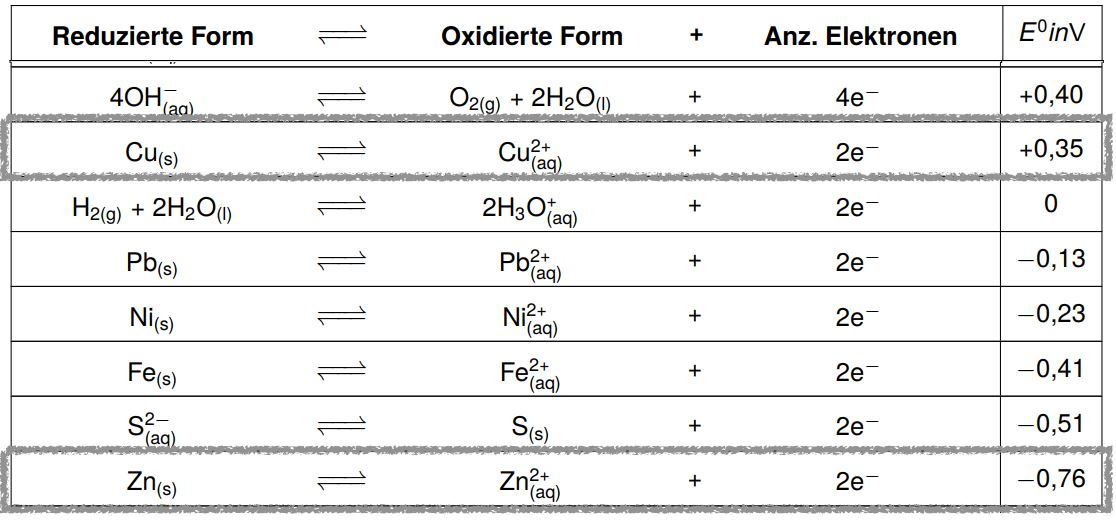Copper is higher than zinc in the table. This means that the reaction to elemental copper takes place earlier than that to elemental zinc, since zinc likes to give up its electrons, but copper does not.

So in general we can say that the reaction takes place when a more noble metal is formed and that it does not take place when a less noble metal is formed.

Note: Sometimes the tables are arranged the other way around, so that the more precious metals are below.

The values ​​of the standard potentials are then not smaller but larger within the table. Then of course the rule applies exactly the other way around!

• An oxidized form, which is higher in the redox series, reacts with a reduced form, which is lower in the redox series.
• An oxidized form, which is lower in the redox series, does NOT react with a reduced form, which is higher.

If we now connect two different half-cells, e.g. a copper and a zinc half-cell, we can also use the electrochemical series to calculate how high the voltage is that can be generated with it. To do this, we subtract the smaller value from the larger: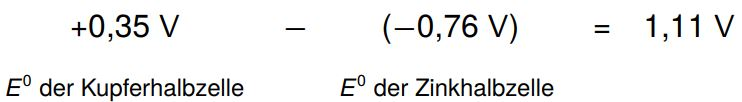The 1.11 V now corresponds to the maximum voltage that can be delivered by this galvanic cell or the minimum voltage that must be applied to reverse the reaction again.